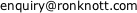Each of the Tests opens in a new window. Tests marked +-*/ link to the Test (in a new window).

All Tests have full Worked Solutions
AS Level Pure Mathematics TESTS.
If you find any bugs or have comments, please email Dr Ron Knott.
For problems with a specific Test, always mention the test reference (a web address) shown in the bottom right hand corner of each Test.

A page to check that this browser correctly displays the maths symbols etc used in these Tests
Powers and Logarithms
Graphs
Sequences and Series
Review of Algebra
Simultaneous Equations
Solving Inequalities
Coordinate Geometry
Trigonometry
Polynomials
Differential Equations
Complex Numbers
Vectors
By adding parameters to the URLs these tests can be particularised to only 2D vector, only 3D vectors, magnitude-direction form only, ij form only, column vectors only and position vectors only. By omitting the parameters, the tests randomly choose the values of the parameters.
eg 2D position vectors for the Magnitude Of Vectors test has URL VectorsMag.html?DIM=2,qu=pts2
Resting your mouse over the URLs below reveals the full URL in the Status bar at the foot of this window.
 2D and 3D Magnitude 2D and 3D magnitude-direction | ij | column vector | position vector Operations 2D and 3D add | subtract | dot (scalar) product | scalar multiple +-*/ Angle between two vectors 2D and 3D Magnitude-direction | ij | column vector | position vector 2D only Magnitude 2D magnitude-direction | ij | column vector | position vector Operations 2D add | subtract | dot (scalar) product | scalar multiple +-*/ Angle between two 2D vectors Magnitude-direction | ij | column vector | position vector Vector Equation of an x-y Line Vector x-y Line to Cartesian form 3D only Magnitude 3D magnitude-direction | ij | column vector | position vector Operations 3D add | subtract | dot (scalar) product | scalar multiple +-*/ Angle between two 3D vectors Magnitude-direction | ij | column vector | position vector Vector Plane Equation using the Normal including 2 zeros, and 1 zero
Matrices
Mechanics
Statistics

Changes
Author : Dr Ron Knott : all enquiries to